Ex 13.5

Chapter 13 Class 12 Probability
Serial order wise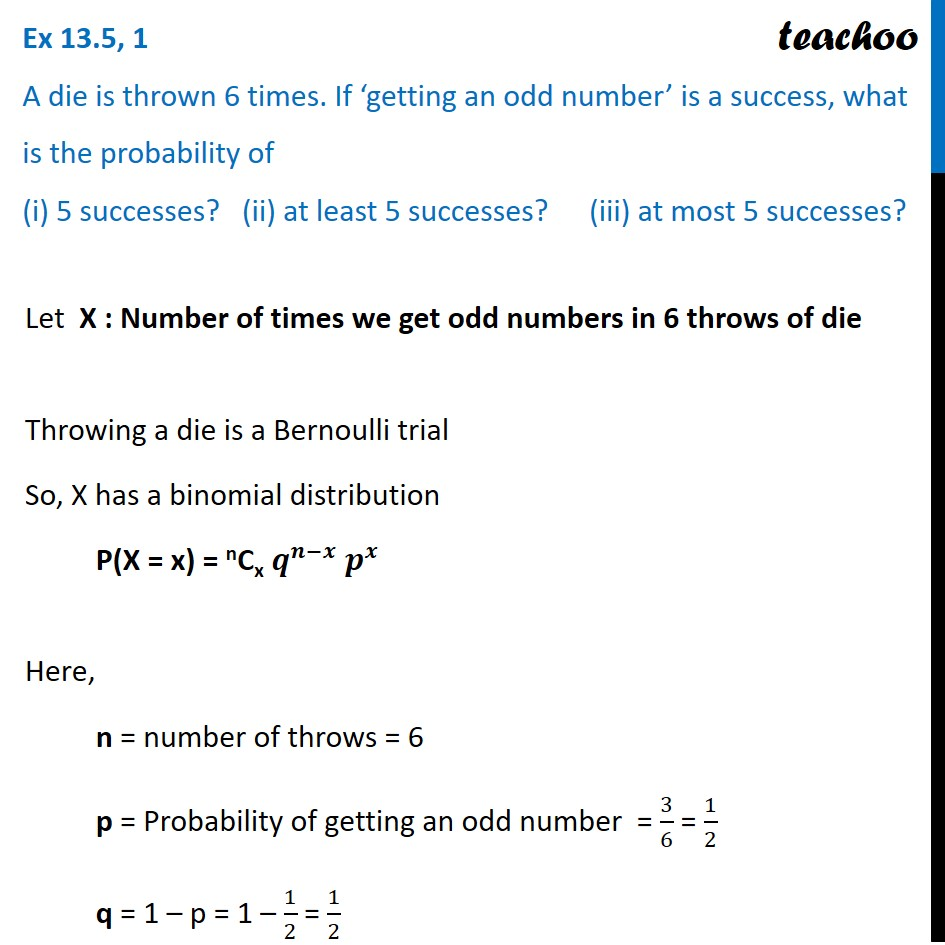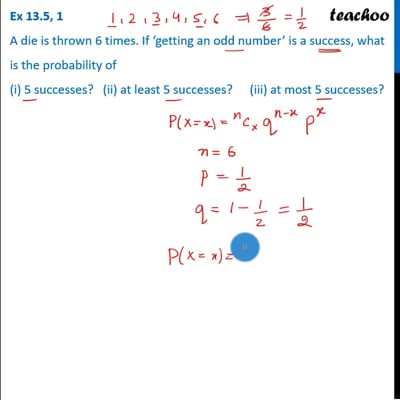This video is only available for Teachoo black users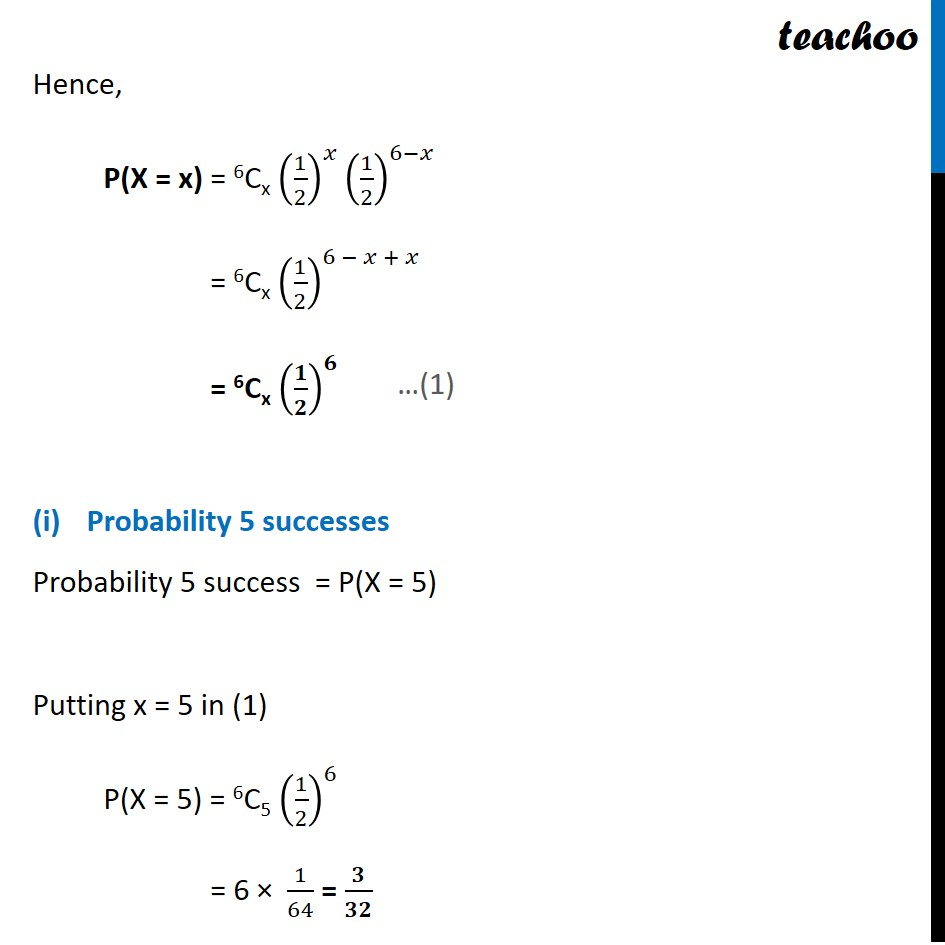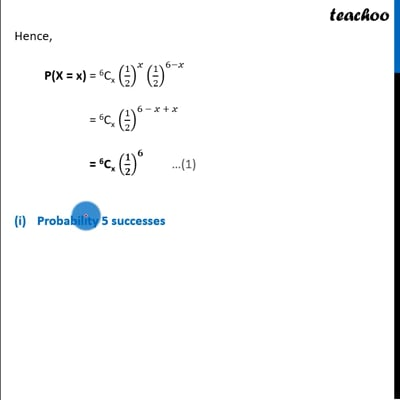This video is only available for Teachoo black users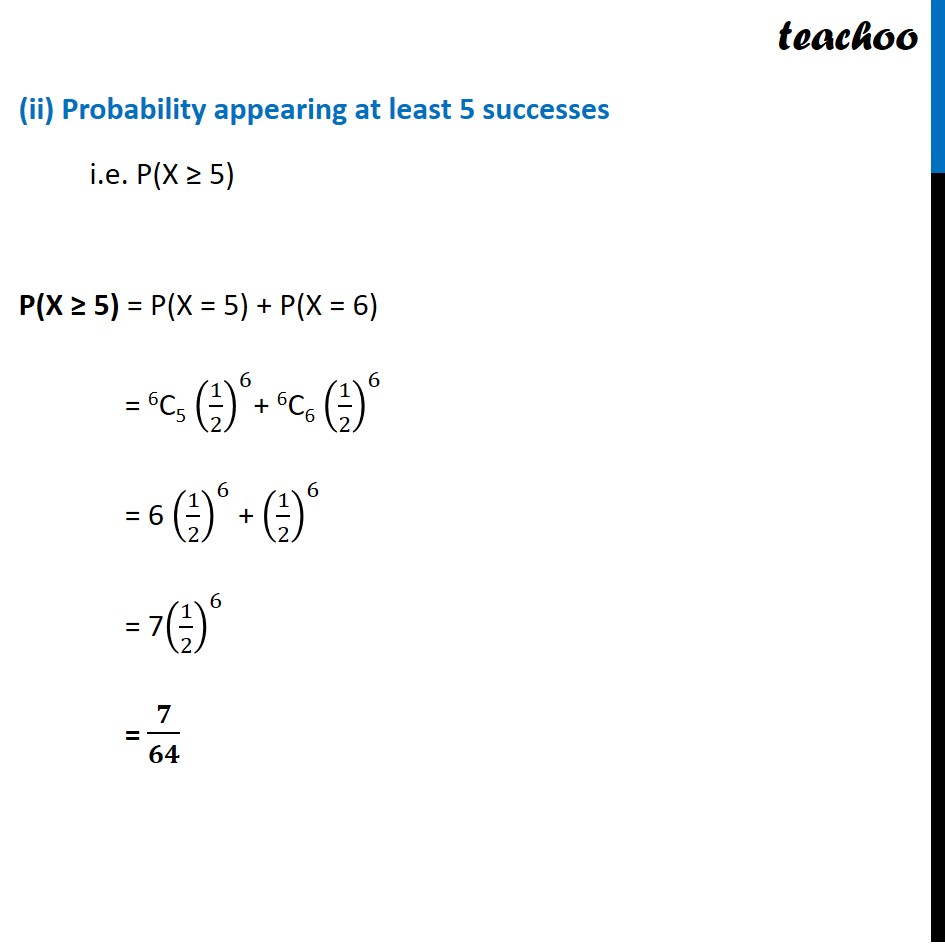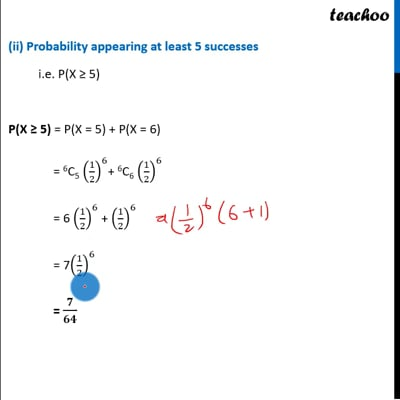This video is only available for Teachoo black users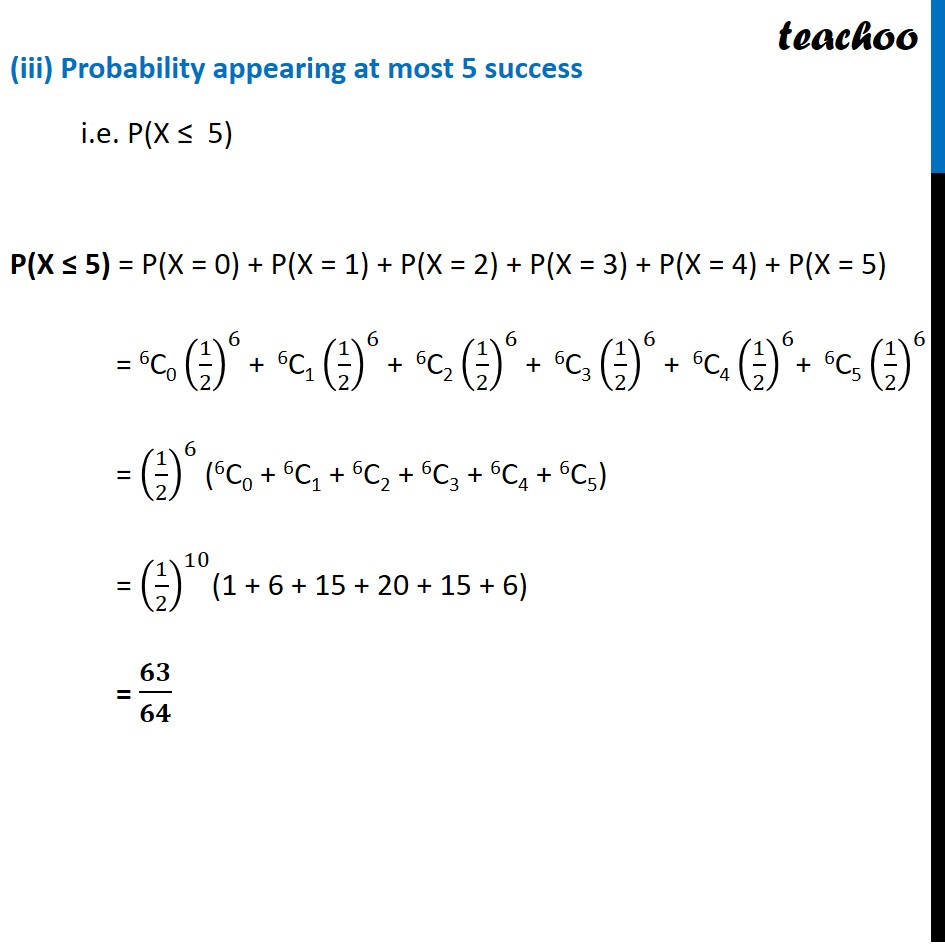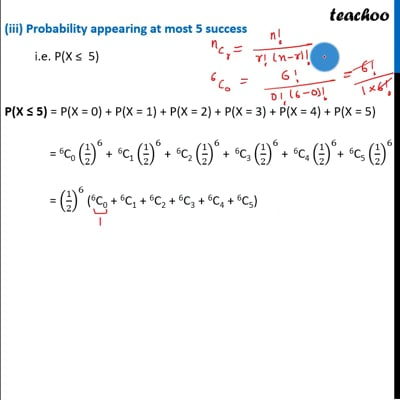This video is only available for Teachoo black users

Solve all your doubts with Teachoo Black (new monthly pack available now!)

### Transcript

Ex 13.5, 1 A die is thrown 6 times. If ‘getting an odd number’ is a success, what is the probability of (i) 5 successes? (ii) at least 5 successes? (iii) at most 5 successes?Let X : Number of times we get odd numbers in 6 throws of die Throwing a die is a Bernoulli trial So, X has a binomial distribution P(X = x) = nCx 𝒒^(𝒏−𝒙) 𝒑^𝒙 Here, n = number of throws = 6 p = Probability of getting an odd number = 3/6 = 1/2 q = 1 – p = 1 – 1/2 = 1/2 Hence, P(X = x) = 6Cx (1/2)^𝑥 (1/2)^(6−𝑥) = 6Cx (1/2)^(6 − 𝑥 + 𝑥) = 6Cx (𝟏/𝟐)^𝟔 Probability 5 successes Probability 5 success = P(X = 5) Putting x = 5 in (1) P(X = 5) = 6C5 (1/2)^6 = 6 × 1/64 = 𝟑/𝟑𝟐 (ii) Probability appearing at least 5 successes i.e. P(X ≥ 5) P(X ≥ 5) = P(X = 5) + P(X = 6) = 6C5 (1/2)^6+ 6C6 (1/2)^6 = 6 (1/2)^6 + (1/2)^6 = 7(1/2)^6 = 𝟕/𝟔𝟒 (iii) Probability appearing at most 5 success i.e. P(X ≤ 5) P(X ≤ 5) = P(X = 0) + P(X = 1) + P(X = 2) + P(X = 3) + P(X = 4) + P(X = 5) = 6C0 (1/2)^6 + 6C1 (1/2)^6 + 6C2 (1/2)^6 + 6C3 (1/2)^6 + 6C4 (1/2)^6+ 6C5 (1/2)^6 = (1/2)^6 (6C0 + 6C1 + 6C2 + 6C3 + 6C4 + 6C5) = (1/2)^10(1 + 6 + 15 + 20 + 15 + 6) = 𝟔𝟑/𝟔𝟒